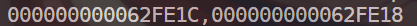# Part 0：为什么要写这篇文章

C语言中的指针是C语言的精髓，也是C语言的重难点之一。

# Part 1：地址和&

1. 小L和小S：我们可以看做是两个变量/常量。
2. 小S的家：这里可以看做是变量/常量小S的地址。
我们要搞清楚，每个变量/常量都和我们一样：我们每个人都有自己的家，正如变量也有自己的地址。通俗的理解，地址是给变量/常量来存放值的地点
3. 路牌：注意注意注意！这里就指出了变量/常量小S的地址：神仙小区403
事实上，我们等会会讲，输出一个变量的地址其实是个16进制的数字。

&这个符号我们一个不陌生，你最初用到应该是在：scanf("%d",&a)里边。
&叫做取址符，用来获取一个变量/常量的地址。

# Part 2：一定要记住的东西

int* ip;            //类型是int*，名称是ip
float* fp;          //类型是float*，名称是fp
double* dp;         //类型是double*，名称是dp


int *ip;
float *fp;
double *dp;


1. 有的初学者会把*p当做是指针名
2. 有的初学者会把定义时出现的*p取值时出现的*p弄混

# Part 3：与指针相关的几个符号

&我们上面讲过，他是来取地址的。举个例子：

#include <stdio.h>
int main(){
int a = 10;
float b = 10.3;
printf("%p,%p",&a,&b);
}


%p用来输出地址，当然，你也可以写成%d或者%x。先不管这个，我们来看看他会输出什么：#include <stdio.h>
int main(){
int a = 10;
int* p;
p = &a;
printf("a的地址：%p\n",&a);
printf("指针p自身的地址：%p\n",&p);
printf("指针p指向的地址：%p",p);
}


a的地址：000000000062FE1C

#### 请注意！如果你的指针为int*，那么你只能指向int类型；如果是double*类型，只能指向double类型，以此类推

*有两个用法。第一个在定义指针时用到，第二个则是取值，什么意思？看下面这个例子：

#include <stdio.h>
int main(){
int a = 10;
int* p;
p = &a;
printf("a的地址：%p\n",&a);
printf("指针p自身的地址：%p\n",&p);
printf("指针p指向的地址：%p\n",p);
printf("指针p指向的地址的值：%d",*p);
}


a的地址：000000000062FE1C

#include <stdio.h>
int main(){
int a = 10;
int* p;
p = &a;
printf("指针p指向的地址的值：%d\n",*p);
*p = 13;
printf("指针p指向的地址的值：%d\n",*p);
*p += 3;
printf("指针p指向的地址的值：%d\n",*p);
*p -= 3;
printf("指针p指向的地址的值：%d\n",*p);
*p *= 9;
printf("指针p指向的地址的值：%d\n",*p);
*p /= 3;
printf("指针p指向的地址的值：%d\n",*p);
*p %= 3;
printf("指针p指向的地址的值：%d\n",*p);
}


# Part 4：交换函数

t = a;
a = b;
b = t;


void swap(int a,int b){
int t;
t = a;
a = b;
b = t;
}


#include <stdio.h>
void swap(int a,int b){
int t;
t = a;
a = b;
b = t;
}
int main(){
int x = 5,y = 10;
printf("x=%d,y=%d\n",x,y);
swap(x,y);
printf("x=%d,y=%d",x,y);
}


x=5,y=10
x=5,y=10

swap(x,y);             --->把x赋值给a，把y赋值给b
///进入函数体
int t;                 --->定义t
t = a;                 --->t赋值为a
a = b;                 --->a赋值为b
b = t;                 --->b赋值为t


void swap(int* a,int* b){
int t;
t = *a;
*a = *b;
*b = t;
}


int a;
&a ---> int*;
double d;
&d ---> double*;
int* p;
&p ---> int**;//这是个二级指针，也就是说指向指针的指针


x=5,y=10
x=10,y=5

# Part 5：char*表示字符串

char*这个神奇的类型可以表示个字符串，举个例子：


#include <stdio.h>

int main()
{
char* str;
str = "YOU AK IOI!";
printf("%s",str);
}


# Part 6：野指针

int* p;
printf("%p",p);


# Part 7：总结

1. 指针是个变量，他的类型是数据类型+*，他的值是一个地址，他自身也有地址
2. 指针有两个专属运算符：&*
3. 指针可以操作变量，不能操作常量
4. 指针可以表示字符串
5. 请注意野指针的问题

1. char[]，char，const char的区别与联系
2. const修饰指针会怎么样？
3. void*指针的运用
4. 多级指针的运用
5. NULL到底是什么
6. malloc函数的运用

posted @ 2020-06-06 10:15  SD！LTF  阅读(486)  评论(2编辑  收藏